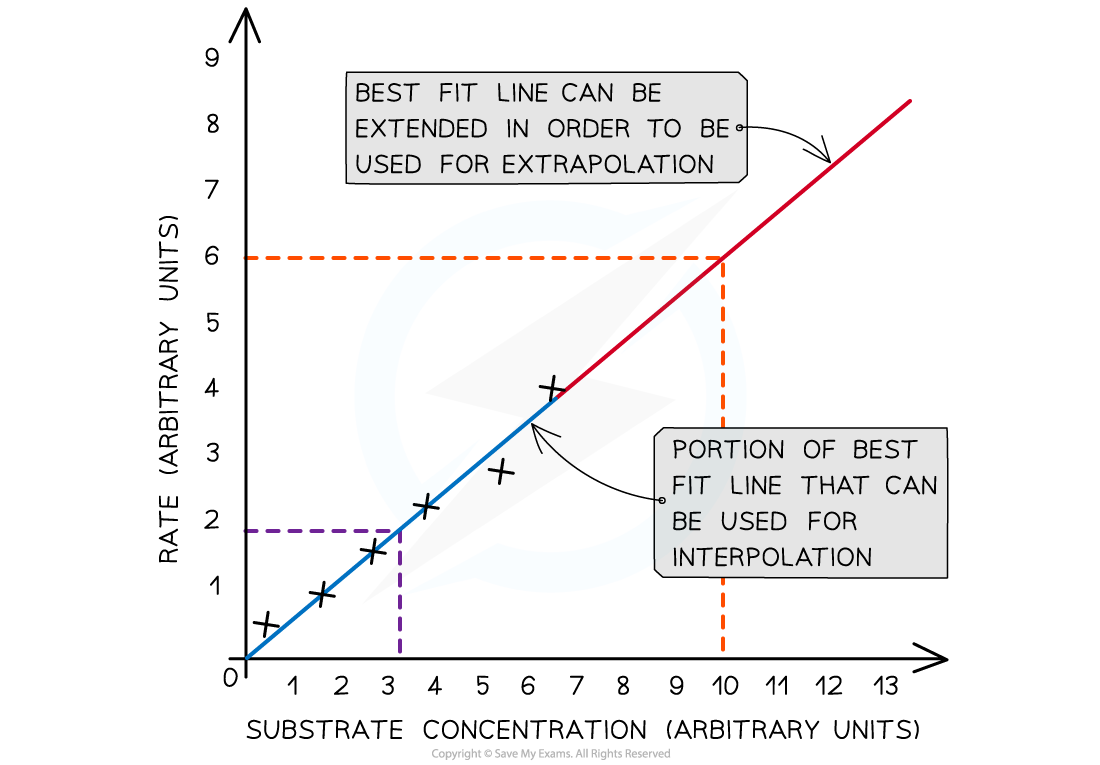# AQA A Level Biology复习笔记1.4.5 Maths Skill: Drawing a Graph for Enzyme Rate Experiments

### Maths Skill: Drawing a Graph for Enzyme Rate Experiments

• Enzyme rate experiments are experiments that are carried out to determine the effect of changing a particular factor on the rate of a reaction that is catalysed by an enzyme
• Factors that can be changed include:
• Temperature
• pH
• Enzyme concentration
• Substrate concentration

• The ways in which the reaction rate can be measured include:
• Measuring how much of a product is made in a given time period (e.g. using a gas cylinder to collect the oxygen produced from the breakdown of hydrogen peroxide by catalase)
• Measuring how much a substrate is broken down in a given time period (e.g. using iodine to determine how quickly starch is broken down into maltose by amylase)

• Line graphs should be used to present the results of enzyme rate experiments
• The data should be plotted with the independent variable on the x-axis and the dependent variable on the y-axis
• If a trend can be identified, a line of best fit (straight or curved) should be added to the graph
• If asked in an exam, you can use this line of best fit to interpolate (reading off values in between existing data points) or extrapolate (going beyond the range of existing data points to read off values)
• Using the graph and best fit line below, for example, you can use interpolation to conclude that, at a substrate concentration of 3 arbitrary units, the rate must have been 1.8 arbitrary units and you can use extrapolation to conclude that, at a substrate concentration of 10 arbitrary units, the rate will be 6 arbitrary unitsThe results of an enzyme rate experiment, with substrate concentration as the independent variable

• For some enzyme rate experiment graphs, it may be necessary to plot more than one set of data on the same graph
• For example, if investigating the effect of temperature on the rate of an enzyme-controlled reaction, you may need to plot a line graph with multiple lines, where each line represents the data collected at a specific temperature
• When drawing a graph like this, make sure to clearly label each line
• An example is shown below:The results of an enzyme experiment. It is the volume of product produced and not the rate of reaction that has been plotted on the y axis for this graph. The initial rate of reaction is represented by the initial gradient of the lines on the graph. As the temperature is the factor being manipulated it is the independent variable.

#### Tips for plotting line graphs

• When plotting line graphs for enzyme rate experiments, remember the following:
• Plot data points accurately
• Use appropriate linear scales on axes
• Choose scales that enable all data points to be plotted within the graph area
• Label axes, with units included
• Make graphs that fill the space the exam paper gives you
• Draw a line (or curve) of best-fit to identify trends. The line must be smooth and have a balance of data points above and below the line
• In some cases, the line of best fit should be drawn through the origin, for example for rate–concentration graphs (the reaction cannot occur if the concentration of enzyme or substrate is 0). The line of best fit should only go through the origin if the data and trend allow it